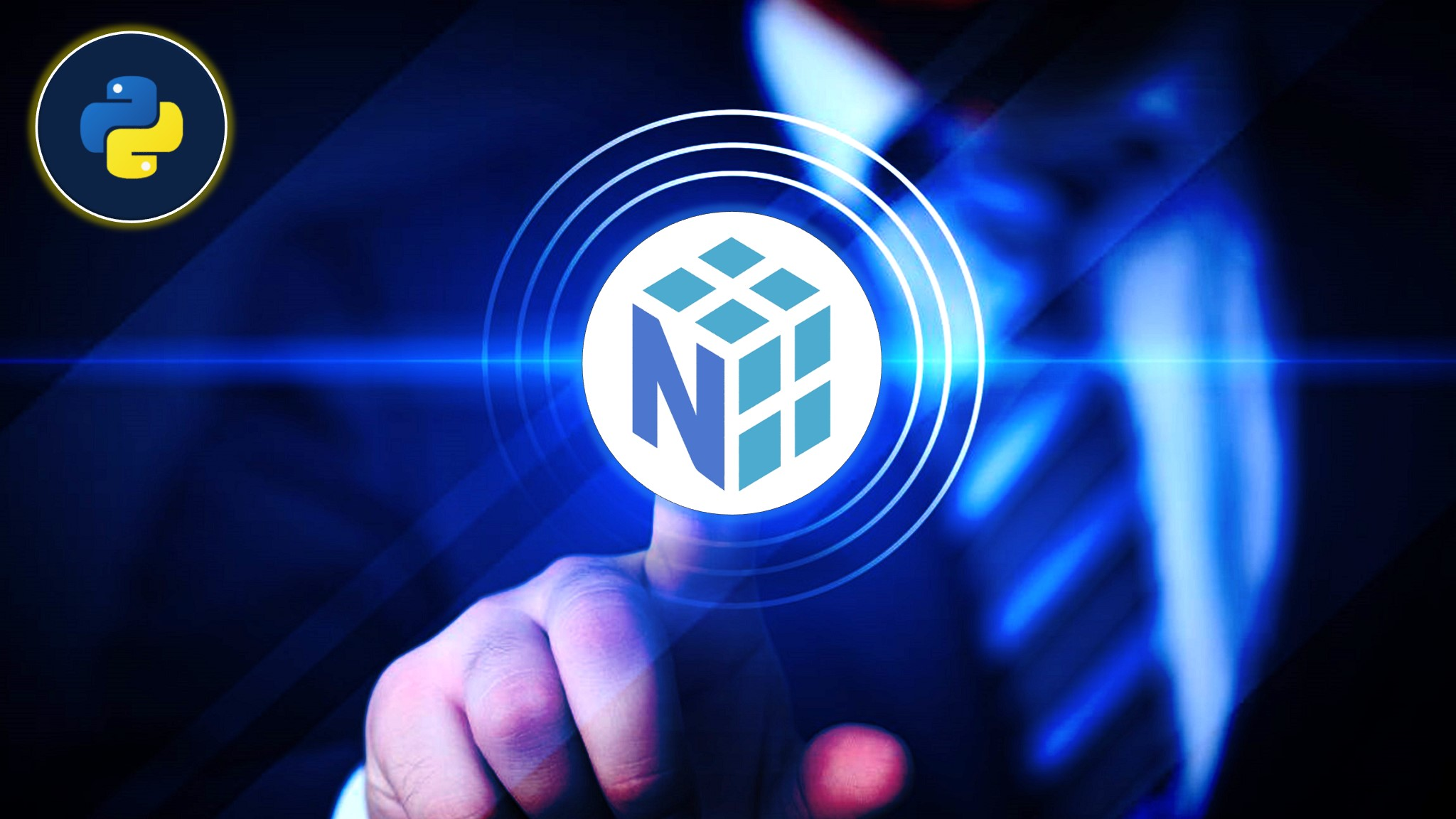# NumPy For Data Science and Machine Learning - Eduonix Free Courses

NumPy is the best tool to learn the fastest python-based numerical computation which is the foundation for many data science packages like pandas for data analysis, sklearn, sci-kit-learn for an ML algorithm.

### Description

Hi, welcome to the 'NumPy For Data Science And Machine Learning' course. This forms the basis for everything else. The central object in Numpy is the Numpy array, on which you can do various operations. We know that the matrix and arrays play an important role in numerical computation and data analysis. Pandas and other ML or AI tools need tabular or array like data to work efficiently, so using NumPy in Pandas and ML packages can reduce the time and improve the performance of the data computation. NumPy based arrays are 10 to 100 times (even more than 100 times) faster than the Python Lists, hence if you are planning to work as a Data Analyst or Data Scientist or Big Data Engineer with Python, then you must be familiar with the NumPy as it offers a more convenient way to work with Matrix like objects like Nd arrays. And also we are going to do a demo where we prove that using a Numpy vectorized operation is faster than normal Python lists.
So if you want to learn about the fastest python based numerical multidimensional data processing framework, which is the foundation for many data science packages like pandas for data analysis, sklearn, scikit-learn for the machine learning algorithm, you are at the right place and right track. The course contents are listed in the "Course content" section of the course, please go through it.
I wish you all the very best and good luck with your future endeavors. Looking forward to seeing you inside the course.

Pruthviraja L
SKILLS YOU WILL GAIN
NumPy Basics and Nd-array creation Numerical Computation Using Python Data Analysis and Extraction Difference between dot product and matrix multiplication WHAT YOU WILL LEARN
NumPy basics How to work with Jupyter notebook as a Data Analyst beginner Python style coding What is Numerical analysis of Data